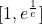# Some results on infinite power towers

Notes on Number Theory and Discrete Mathematics, ISSN 1310-5132
Volume 16, 2010, Number 3, Pages 18—24

## Details

### Authors and affiliations

Mladen Vassilev-Missana5, V. Hugo Str., Sofia-1124, Bulgaria

### Abstract

In the paper the infinite power towers which are generated by an algebraic numbers belonging to the closed intervalare investigated and an answer is given to the question when they are transcendental or rational numbers. Also a necessary condition for an infinite power tower to be an irrational algebraic number is proposed.

### Keywords

• Infinite power tower
• Algebraic number
• Transcendental number

### References

1. Baker, A. Transcendental Number Theory. London, Cambridge University Press, 1990.
2. Vassilev-Missana, M. A short remark on transcendental numbers. Notes on Number Theory and Discrete Mathematics, Vol. 14, No. 4, 2008, 1-3.
3. Shkliarski D., N. Chentzov, I. Yaglom. The USSR Olympiad Problem Book: Selected Problems and Theorems of Elementary Mathematics, New York, Dover Publications, 1993.

## Related papers

1. Ripà, M. (2020). On the constant congruence speed of tetration, Notes on Number Theory and Discrete Mathematics, 26(3), 245–260.
2. Ripà, M. (2021). The congruence speed formula. Notes on Number Theory and Discrete Mathematics, 27(4), 43-61.
3. Ripà, M., & Onnis, L. (2022). Number of stable digits of any integer tetration. Notes on Number Theory and Discrete Mathematics, 28(3), 441-457.

## Cite this paper

Vassilev-Missana, M. (2010). Some results on infinite power towers. Notes on Number Theory and Discrete Mathematics, 16(3), 18-24.